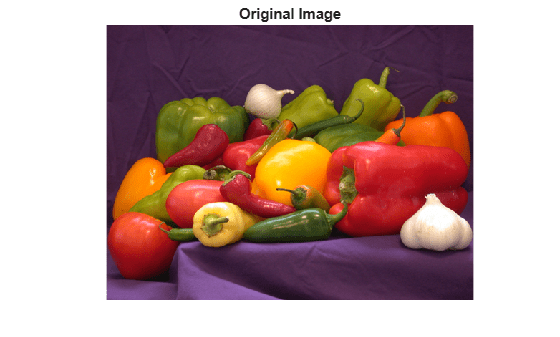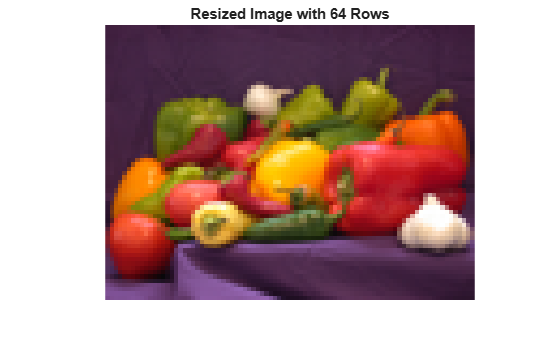# imresize

## Syntax

``B = imresize(A,scale)``
``B = imresize(A,[numrows numcols])``
``````[Y,newmap] = imresize(X,map,___)``````
``___ = imresize(___,method)``
``___ = imresize(___,Name,Value)``

## Description

example

````B = imresize(A,scale)` returns image `B` that is `scale` times the size of image `A`. The input image `A` can be a grayscale, RGB, binary, or categorical image.If `A` has more than two dimensions, then `imresize` only resizes the first two dimensions. If `scale` is between 0 and 1, then `B` is smaller than `A`. If `scale` is greater than 1, then `B` is larger than `A`. By default, `imresize` uses bicubic interpolation.```

example

````B = imresize(A,[numrows numcols])` returns image `B` that has the number of rows and columns specified by the two-element vector `[numrows numcols]`. ```

example

``````[Y,newmap] = imresize(X,map,___)``` resizes the indexed image `X` where `map` is the colormap associated with the image.```

example

````___ = imresize(___,method)` specifies the interpolation method used.```
````___ = imresize(___,Name,Value)` returns the resized image where name-value arguments control various aspects of the resizing operation. Specify name-value arguments after all other input arguments.```

## Examples

collapse all

`I = imread('ngc6543a.jpg');`

Shrink the image by a factor of two.

`J = imresize(I, 0.5);`

Display the original image and the resized image.

`figure, imshow(I), figure, imshow(J)`Load an image into the workspace.

`I = imread('ngc6543a.jpg');`

Shrink the image to 40% of the original size using nearest-neighbor interpolation. This is the fastest method, but it has the lowest quality.

`J = imresize(I,0.4,'nearest');`

Display the original image and the resized image.

```imshow(I) title('Original Image')``````imshow(J) title('Resized Image Using Nearest Neighbor Interpolation')```Read an RGB image into the workspace.

`RGB = imread('peppers.png');`

Resize the RGB image to have 64 rows. `imresize` calculates the number of columns automatically.

`RGB2 = imresize(RGB,[64 NaN]);`

Get the size of the resized image.

`sz = size(RGB2)`
```sz = 1×3 64 86 3 ```

Display the original image and the resized image.

```imshow(RGB) title("Original Image")``````imshow(RGB2) title("Resized Image with 64 Rows")```Read an indexed image into the workspace.

`[X,map] = imread("corn.tif");`

Increase the size of the indexed image by 50%.

`[Y,newmap] = imresize(X,map,1.5);`

Display the original image and the resized image.

```imshow(X,map) title("Original Indexed Image and Colormap")``````imshow(Y,newmap) title("Resized Image and Optimized Colormap")```## Input Arguments

collapse all

Image to be resized, specified as a numeric array, logical array, or categorical array of any dimension. Input must be nonsparse, and numeric input must be real.

Data Types: `single` | `double` | `int8` | `int16` | `int32` | `uint8` | `uint16` | `uint32` | `logical` | `categorical`

Resize factor, specified as a positive number. `imresize` applies the same scale factor to the row and column dimensions. To apply a different resize factor to each dimension, use the `Scale` name-value argument.

Data Types: `single` | `double` | `int8` | `int16` | `int32` | `int64` | `uint8` | `uint16` | `uint32` | `uint64`

Row and column dimensions of output image, specified as a two-element vector of positive numbers. You can specify the value `NaN` for either `numrows` or `numcols`. In this case, `imresize` calculates the number of rows or columns for that dimension automatically, preserving the aspect ratio of the image.

Data Types: `single` | `double` | `int8` | `int16` | `int32` | `int64` | `uint8` | `uint16` | `uint32` | `uint64`

Indexed image to be resized, specified as a real, nonsparse numeric array of positive integers.

Data Types: `double` | `uint8` | `uint16`

Colormap associated with indexed image `X`, specified as a c-by-3 numeric matrix with values in the range [0, 1]. Each row is a three-element RGB triplet that specifies the red, green, and blue components of a single color of the colormap.

Data Types: `double`

Interpolation method, specified as a character vector, string scalar, or two-element cell array. The default value for numeric and logical images is `"bicubic"`. The default value for categorical images is `"nearest"`. Categorical images only support the values `"nearest"` and `"box"`.

When `method` is a character vector or string scalar, it identifies a particular method or named interpolation kernel, listed in the following table.

MethodDescription

`"nearest"`

Nearest-neighbor interpolation; the output pixel is assigned the value of the pixel that the point falls within. No other pixels are considered.

`"bilinear"`

Bilinear interpolation; the output pixel value is a weighted average of pixels in the nearest 2-by-2 neighborhood

`"bicubic"`

Bicubic interpolation; the output pixel value is a weighted average of pixels in the nearest 4-by-4 neighborhood.

Note

Bicubic interpolation can produce pixel values outside the original range.

Interpolation KernelDescription
`"box"`

Box-shaped kernel

`"triangle"`Triangular kernel (equivalent to `"bilinear"`)
`"cubic"`Cubic kernel (equivalent to `"bicubic"`)
`"lanczos2"`Lanczos-2 kernel
`"lanczos3"`Lanczos-3 kernel

When `method` is a two-element cell array, it defines a custom interpolation kernel. The cell array has the form {f,w}, where f is a function handle for a custom interpolation kernel and w is the width of the custom kernel. f(x) must be zero outside the interval -w/2 <= x < w/2. The function handle f can be called with a scalar or a vector input. For user-specified interpolation kernels, the output image can have some values slightly outside the range of pixel values in the input image.

For more information about the built-in and custom interpolation kernels, see Create and Compare Resizing Interpolation Kernels.

Data Types: `char` | `string` | `cell`

### Name-Value Arguments

Specify optional pairs of arguments as `Name1=Value1,...,NameN=ValueN`, where `Name` is the argument name and `Value` is the corresponding value. Name-value arguments must appear after other arguments, but the order of the pairs does not matter.

Example: `B = imresize(A,0.5,Antialiasing=false)` does not perform antialiasing when shrinking an image.

Before R2021a, use commas to separate each name and value, and enclose `Name` in quotes.

Example: `B = imresize(A,0.5,"Antialiasing",false)` does not perform antialiasing when shrinking an image.

Perform antialiasing when shrinking an image, specified as `true` or `false`.

• If the interpolation `method` is `"nearest"`, then the default value of `Antialiasing` is `false`.

• If the interpolation `method` is `"box"` and the input image is categorical, then the default value of `Antialiasing` is `false`.

• For all other interpolation methods, the default value is `true`.

Data Types: `logical`

Return optimized or original colormap for indexed image, specified as one of the following values.

ValueDescription
`"original"`The output colormap `newmap` is the same as the input colormap `map`.
`"optimized"``imresize` returns a new optimized colormap.

The `Colormap` argument is valid only when resizing indexed images.

Data Types: `char` | `string`

Perform color dithering, specified as `true` or `false`. In dithering, you apply a form of noise to the image to randomize quantization error and prevent large-scale patterns.

The `Dither` argument is valid only when resizing indexed images.

Data Types: `logical`

Interpolation method, specified as a character vector, string scalar, or two-element cell array. For details, see `method`.

Data Types: `char` | `string` | `cell`

Size of the output image, specified as a two-element vector of positive numbers. For details, see ```[numrows numcols]```.

Data Types: `single` | `double` | `int8` | `int16` | `int32` | `int64` | `uint8` | `uint16` | `uint32` | `uint64`

Resize scale factor, specified as a positive number or two-element vector of positive numbers. If you specify a scalar, then `imresize` applies the same scale factor to the row and column dimensions. If you specify a two-element vector, then `imresize` applies a different scale value to each dimension.

Data Types: `single` | `double` | `int8` | `int16` | `int32` | `int64` | `uint8` | `uint16` | `uint32` | `uint64`

## Output Arguments

collapse all

Resized image, returned as a numeric, logical, or categorical array of the same data type as the input image, `A`.

Resized indexed image, returned as a numeric array of the same data type as the input indexed image, `X`.

Colormap of the resized indexed image `Y`, returned as an m-by-3 numeric matrix. By default, `imresize` returns a new, optimized colormap with the resized image. To return a colormap that is the same as the original colormap, use the `"Colormap"` name-value argument.

## Tips

• If the size of the output image is not an integer, then `imresize` does not use the scale specified. `imresize` uses the `ceil` function when calculating the output image size.

## Version History

Introduced before R2006a

expand all